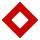Diamond

Calculate the length of the two diagonals of the diamond if:

a = 13 cm
v = 12 cm

Result

u1 =  21.63 cm
u2 =  14.42 cm

Solution:Leave us a comment of example and its solution (i.e. if it is still somewhat unclear...):Be the first to comment!To solve this verbal math problem are needed these knowledge from mathematics:

Pythagorean theorem is the base for the right triangle calculator.

Next similar examples:

1. DiamondSide length of diamond is 35 cm and the length of the diagonal is 56 cm. Calculate the height and length of the second diagonal.
2. Diagonals in diamons/rhombusRhombus ABCD has side length AB = 4 cm and a length of one diagonal of 6.4 cm. Calculate the length of the other diagonal.
3. DiamondDetermine the side of diamond if its content is S = 353 cm2 and one diagonal u2 = 45 cm.
4. TetrahedronCalculate height and volume of a regular tetrahedron whose edge has a length 18 cm.
5. DiagonalsA diagonal of a rhombus is 20 cm long. If it's one side is 26 cm find the length of the other diagonal.
6. SatinSanusha buys a piece of satin 2.4 m wide. The diagonal length of the fabric is 4m. What is the length of the piece of satin?
7. UmbrellaCan umbrella 75 cm long fit into a box of fruit? The box has dimensions of 390 mm and 510 mm.
8. Theorem proveWe want to prove the sentence: If the natural number n is divisible by six, then n is divisible by three. From what assumption we started?
9. Functions f,gFind g(1) if g(x) = 3x - x2 Find f(5) if f(x) = x + 1/2
10. Factory and divisionsThe factory consists of three auxiliary divisions total 2,406 employees. The second division has 76 employees less than 1st division and 3rd division has 212 employees more than the 2nd. How many employees has each division?
11. PowersExpress the expression ? as the n-th power of the base 10.
12. Nineteenth memberFind the nineteenth member of the arithmetic sequence: a1=33 d=5 find a19
13. 6 termsFind the first six terms of the sequence. a1 = 7, an = an-1 + 6
14. Linear systemSolve a set of two equations of two unknowns: 1.5x+1.2y=0.6 0.8x-0.2y=2
15. TreesA certain species of tree grows an average of 0.5 cm per week. Write an equation for the sequence that represents the weekly height of this tree in centimeters if the measurements begin when the tree is 200 centimeters tall.
16. Volleyball8 girls wants to play volleyball against boys. On the field at one time can be six players per team. How many initial teams of this girls may trainer to choose?
17. One halfOne half of ? is: ?